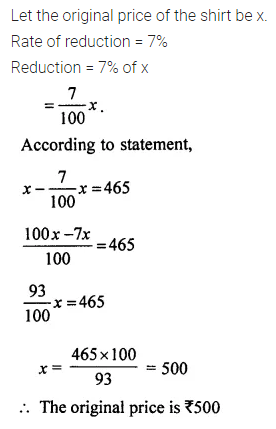# ML Aggarwal Class 7 Solutions for ICSE Maths Chapter 7 Percentage and Its Applications Ex 7.2

## ML Aggarwal Class 7 Solutions for ICSE Maths Chapter 7 Percentage and Its Applications Ex 7.2

Question 1.
Find:
(i) 15% of 250
(ii) 25% of 120 litres
(iii) 1% of 1 hour
(iv) 75% of 1 kg
(v) 120% of ₹ 250
(vi) 0.6% of 2 km
Solution: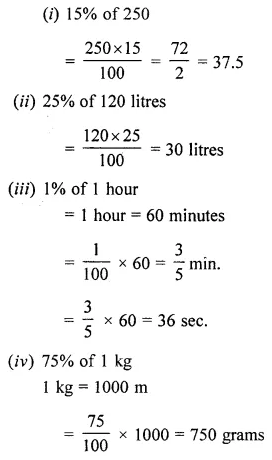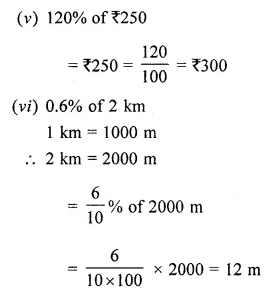Question 2.
8% of children of a class of 25 like getting wet in the rain. How many children like getting wet in the rain?
Solution:Question 3.
Vasundhara ate 3 ice cream cups out of 20 kept in the fridge. What per cent did she eat?
Solution: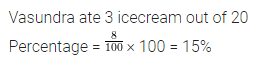Question 4.
Express:
(i) 20 as a percentage of 50
(ii) 60 litres as a percentage of 40 litres
(iii) 90 cm as a percentage of 4.5 m
(iv) 350 g as a percentage of 5.6 kg
Solution:Question 5.
What per cent is:
(i) 12 of 80
(ii) 25 paise of 4 rupees
(iii) 300 g of 2 kg
Solution: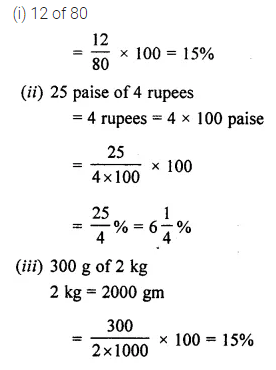Question 6.
A school team won 6 games this year against 4 games won last year. What is the per cent increase?
Solution:Question 7.
The price of a shirt decreased from ₹ 80 to ₹ 60, find the percentage of decrease in the price of the shirt.
Solution:Question 8.
My mother says, in her childhood petrol was ₹ 1 per litre. It is ₹ 65 per litre today. By what percentage has the prices of petrol gone up?
Solution: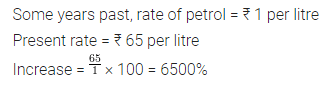Question 9.
Rate of basmati rice last year was ₹ 40 a kg. This year they are costly by 20%. What is the price this year?
Solution: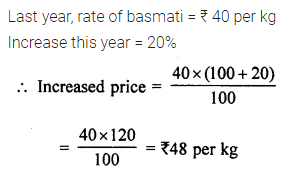Question 10.
300 students took an exam. 28% failed. Calculate the number of students who passed the exam.
Solution: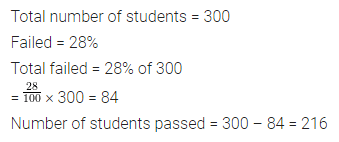Question 11.
Out of 15000 voters in a constituency, 60% voted. Find the number of voters who did not vote.
Solution:Question 12.
20% of the length of a flagpole is painted green, 45% is painted yellow and the remaining red. If the length of the pole is 18 m, what length of it is painted red?
Solution: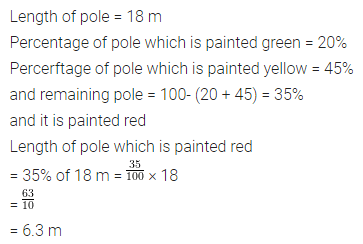Question 13.
Chalk contains 10% calcium, 3% carbon, 12% oxygen and the remaining sand. Find the amount of carbon and calcium (in grams) in 2$$\frac { 1 }{ 2 }$$ kg of chalk. Also, find the amount of sand (in kg).
Solution: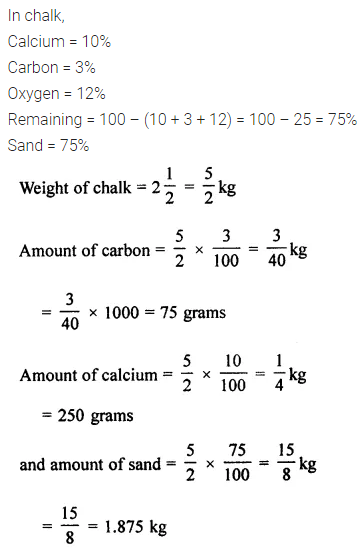Question 14.
Find the whole quantity if:
(i) 25% of it is 9
(ii) 75% of it is 15
(iii) 12% of it is ₹ 1080
(iv) 8% of it is 40 litres
Solution: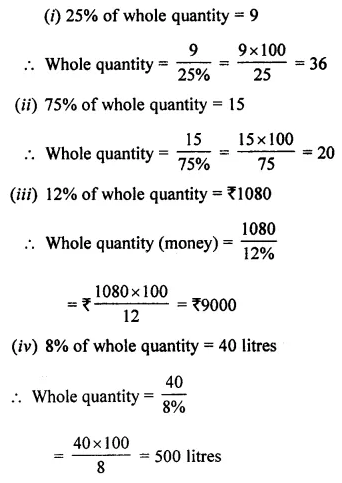Question 15.
Mohini saves ₹ 400 from her salary. If this is 10% of her salary, then what is her salary?
Solution:Question 16.
16% of the apples in a basket go bad. If there are 42 good apples in the basket, find the total number of apples in the basket.
Solution: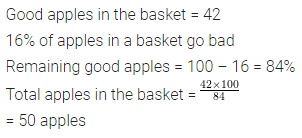Question 17.
In an examination, a student has to secure 45% marks to pass the exam. If Varun got 251 marks and failed by 19 marks, what are the maximum marks?
Solution: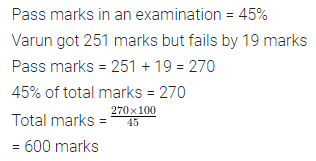Question 18.
On a rainy day, 94% of the students were students absent on that day was 174, find the total strength of the school.
Solution: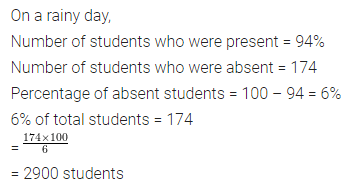Question 19.
40% of the population of a town are men and 39% are women. If the number of children is 12600, find the number of men.
Solutions:Question 20.
If the price of a watch is increased by 15%, the increase in the price is ₹ 90. What was the price of watching earlier?
Solution:Question 21.
(i) Find the number which when increased by 30% becomes 39.
(ii) Find the number which when decreased by 8% becomes 506.
Solution:Question 22.
The price of a shirt is reduced by 7% to ₹ 465. What is its original price?
Solution: